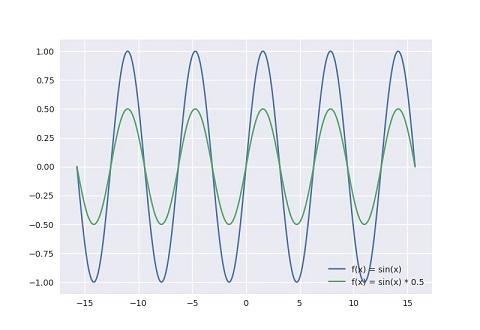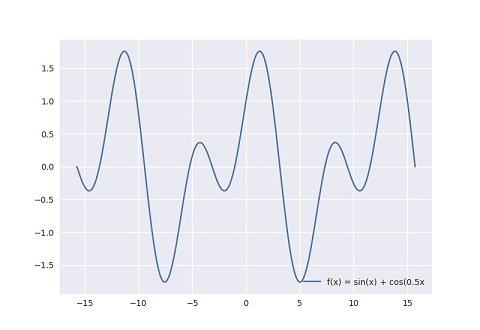# GB/音频/声音的构成

• 响度: 声音的大小, 决定于物体震动幅度大小(即振幅大小).
• 音调: 声音的高低, 決定于物体震动速度快慢(即频率高低).
• 音色: 声音的特色, 決定于声音的波形.

## 响度## 音调## 音色## 关于如何绘制波形图

``````import numpy as np
import matplotlib.pyplot as plt
plt.style.use('seaborn')
plt.rcParams.update({'font.size': 22})

ax = plt.subplot()

x = np.linspace(-5*np.pi, 5*np.pi, 256)
ax.plot(x, np.sin(x), label='f(x) = sin(x)')

x = np.linspace(-5*np.pi, 5*np.pi, 256)
ax.plot(x, 0.5 * np.sin(x), label='f(x) = sin(x) * 0.5')

ax.legend(loc='lower right')
plt.show()
``````
``````import numpy as np
import matplotlib.pyplot as plt
plt.style.use('seaborn')
plt.rcParams.update({'font.size': 22})

ax = plt.subplot()

x = np.linspace(-3*np.pi, 3*np.pi, 256)
ax.plot(x, np.sin(x), label='f(x) = sin(x)')

x = np.linspace(-3*np.pi, 3*np.pi, 256)
ax.plot(x, np.sin(3 * x), label='f(x) = sin(3x)')

ax.legend(loc='lower right')
plt.show()
``````
``````import numpy as np
import matplotlib.pyplot as plt
plt.style.use('seaborn')
plt.rcParams.update({'font.size': 22})

ax = plt.subplot()

x = np.linspace(-5*np.pi, 5*np.pi, 256)
ax.plot(x, np.sin(x) + np.cos(0.5 * x), label='f(x) = sin(x) + cos(0.5x')

ax.legend(loc='lower right')
plt.show()
``````